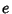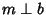## e-Perfect Number

A numberis called an-perfect number if, whereis the Sum of the e-Divisor of. Ifis Squarefree, then. As a result, ifis-perfect andis Squarefree with, thenis-perfect. There are no Odd-perfect numbers.

Guy, R. K. Exponential-Perfect Numbers.'' §B17 in Unsolved Problems in Number Theory, 2nd ed. New York: Springer-Verlag, p. 73, 1994.
Subbarao, M. V. and Suryanarayan, D. Exponential Perfect and Unitary Perfect Numbers.'' Not. Amer. Math. Soc. 18, 798, 1971.